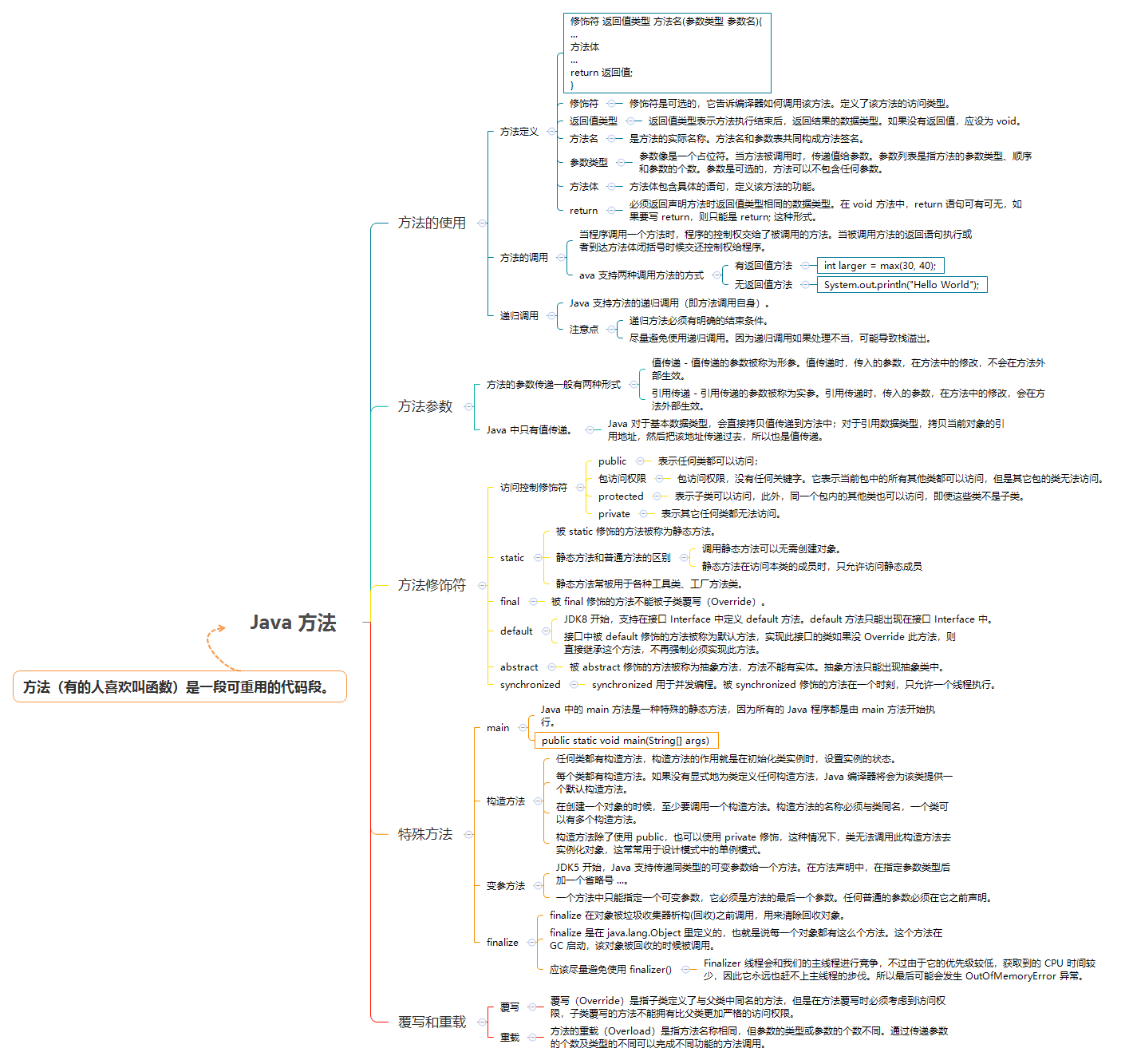# # 深入理解 Java 方法

📦 本文以及示例源码已归档在 javacore (opens new window)

## # 1. 方法的使用

### # 1.1. 方法定义

``````[修饰符] 返回值类型 方法名([参数类型 参数名]){
...
方法体
...
return 返回值;
}
``````

``````public static void main(String[] args) {
System.out.println("Hello World");
}
``````

• 修饰符 - 修饰符是可选的，它告诉编译器如何调用该方法。定义了该方法的访问类型。
• 返回值类型 - 返回值类型表示方法执行结束后，返回结果的数据类型。如果没有返回值，应设为 void。
• 方法名 - 是方法的实际名称。方法名和参数表共同构成方法签名。
• 参数类型 - 参数像是一个占位符。当方法被调用时，传递值给参数。参数列表是指方法的参数类型、顺序和参数的个数。参数是可选的，方法可以不包含任何参数。
• 方法体 - 方法体包含具体的语句，定义该方法的功能。
• return - 必须返回声明方法时返回值类型相同的数据类型。在 void 方法中，return 语句可有可无，如果要写 return，则只能是 `return;` 这种形式。

### # 1.2. 方法的调用

Java 支持两种调用方法的方式，根据方法是否有返回值来选择。

• 有返回值方法 - 有返回值方法通常被用来给一个变量赋值或代入到运算表达式中进行计算。
``````int larger = max(30, 40);
``````
• 无返回值方法 - 无返回值方法只能是一条语句。
``````System.out.println("Hello World");
``````

#### # 递归调用

Java 支持方法的递归调用（即方法调用自身）。

🔔 注意：

• 递归方法必须有明确的结束条件。
• 尽量避免使用递归调用。因为递归调用如果处理不当，可能导致栈溢出。

``````public class RecursionMethodDemo {
public static int fib(int num) {
if (num == 1 || num == 2) {
return 1;
} else {
return fib(num - 2) + fib(num - 1);
}
}

public static void main(String[] args) {
for (int i = 1; i < 10; i++) {
System.out.print(fib(i) + "\t");
}
}
}
``````

## # 2. 方法参数

• 值传递 - 值传递的参数被称为形参。值传递时，传入的参数，在方法中的修改，不会在方法外部生效。
• 引用传递 - 引用传递的参数被称为实参。引用传递时，传入的参数，在方法中的修改，会在方法外部生效。

Java 中只有值传递。

``````public class MethodParamDemo {
public static void method(int value) {
value =  value + 1;
}
public static void main(String[] args) {
int num = 0;
method(num);
System.out.println("num = [" + num + "]");
method(num);
System.out.println("num = [" + num + "]");
}
}
// Output:
// num = 
// num = 
``````

``````public class MethodParamDemo2 {
public static void method(StringBuilder sb) {
sb = new StringBuilder("B");
}

public static void main(String[] args) {
StringBuilder sb = new StringBuilder("A");
System.out.println("sb = [" + sb.toString() + "]");
method(sb);
System.out.println("sb = [" + sb.toString() + "]");
sb = new StringBuilder("C");
System.out.println("sb = [" + sb.toString() + "]");
}
}
// Output:
// sb = [A]
// sb = [A]
// sb = [C]
``````

Java 对于基本数据类型，会直接拷贝值传递到方法中；对于引用数据类型，拷贝当前对象的引用地址，然后把该地址传递过去，所以也是值传递。

## # 3. 方法修饰符

Java 方法有好几个修饰符，让我们一一来认识一下：

### # 3.1. 访问控制修饰符

``````public > protected > 包访问权限（没有任何关键字）> private
``````
• `public` - 表示任何类都可以访问；
• `包访问权限` - 包访问权限，没有任何关键字。它表示当前包中的所有其他类都可以访问，但是其它包的类无法访问。
• `protected` - 表示子类可以访问，此外，同一个包内的其他类也可以访问，即使这些类不是子类。
• `private` - 表示其它任何类都无法访问。

### # 3.2. static

`static` 修饰的方法被称为静态方法。

• 在外部调用静态方法时，可以使用 `类名.方法名` 的方式，也可以使用 `对象名.方法名` 的方式。而实例方法只有后面这种方式。也就是说，调用静态方法可以无需创建对象

• 静态方法在访问本类的成员时，只允许访问静态成员（即静态成员变量和静态方法），而不允许访问实例成员变量和实例方法；实例方法则无此限制。

### # 3.3. final

`final` 修饰的方法不能被子类覆写（Override）。

final 方法示例：

``````public class FinalMethodDemo {
static class Father {
protected final void print() {
System.out.println("call Father print()");
};
}

static class Son extends Father {
@Override
protected void print() {
System.out.println("call print()");
}
}

public static void main(String[] args) {
Father demo = new Son();
demo.print();
}
}
// 编译时会报错
``````

### # 3.4. default

JDK8 开始，支持在接口 `Interface` 中定义 `default` 方法。`default` 方法只能出现在接口 `Interface`

default 方法语法的出现，是为了既有的成千上万的 Java 类库的类增加新的功能， 且不必对这些类重新进行设计。 举例来说，JDK8 中 `Collection` 类中有一个非常方便的 `stream()` 方法，就是被修饰为 `default`，Collection 的一大堆 List、Set 子类就直接继承了这个方法 I，不必再为每个子类都注意添加这个方法。

`default` 方法示例：

``````public class DefaultMethodDemo {
interface MyInterface {
default void print() {
System.out.println("Hello World");
}
}

static class MyClass implements MyInterface {}

public static void main(String[] args) {
MyInterface obj = new MyClass();
obj.print();
}
}
// Output:
// Hello World
``````

### # 3.5. abstract

`abstract` 修饰的方法被称为抽象方法，方法不能有实体。抽象方法只能出现抽象类中。

``````public class AbstractMethodDemo {
static abstract class AbstractClass {
abstract void print();
}

static class ConcreteClass extends AbstractClass {
@Override
void print() {
System.out.println("call print()");
}
}

public static void main(String[] args) {
AbstractClass demo = new ConcreteClass();
demo.print();
}

}
// Outpu:
// call print()
``````

### # 3.6. synchronized

`synchronized` 用于并发编程。`synchronized` 修饰的方法在一个时刻，只允许一个线程执行。

## # 4. 特殊方法

Java 中，有一些较为特殊的方法，分别使用于特殊的场景。

### # 4.1. main 方法

Java 中的 main 方法是一种特殊的静态方法，因为所有的 Java 程序都是由 `public static void main(String[] args)` 方法开始执行。

``````public class MainMethodDemo {
public static void main(String[] args) {
for (String arg : args) {
System.out.println("arg = [" + arg + "]");
}
}
}
``````

``````javac MainMethodDemo.java
java MainMethodDemo A B C
``````

``````arg = [A]
arg = [B]
arg = [C]
``````

### # 4.2. 构造方法

``````public class ConstructorMethodDemo {

static class Person {
private String name;

public Person(String name) {
this.name = name;
}

public String getName() {
return name;
}

public void setName(String name) {
this.name = name;
}
}

public static void main(String[] args) {
Person person = new Person("jack");
System.out.println("person name is " + person.getName());
}
}
``````

### # 4.3. 变参方法

JDK5 开始，Java 支持传递同类型的可变参数给一个方法。在方法声明中，在指定参数类型后加一个省略号 `...`。一个方法中只能指定一个可变参数，它必须是方法的最后一个参数。任何普通的参数必须在它之前声明。

``````public class VarargsDemo {
public static void method(String... params) {
System.out.println("params.length = " + params.length);
for (String param : params) {
System.out.println("params = [" + param + "]");
}
}

public static void main(String[] args) {
method("red");
method("red", "yellow");
method("red", "yellow", "blue");
}
}
// Output:
// params.length = 1
// params = [red]
// params.length = 2
// params = [red]
// params = [yellow]
// params.length = 3
// params = [red]
// params = [yellow]
// params = [blue]
``````

### # 4.4. finalize() 方法

`finalize` 在对象被垃圾收集器析构(回收)之前调用，用来清除回收对象。

`finalize` 是在 `java.lang.Object` 里定义的，也就是说每一个对象都有这么个方法。这个方法在 GC 启动，该对象被回收的时候被调用。

finalizer() 通常是不可预测的，也是很危险的，一般情况下是不必要的。使用终结方法会导致行为不稳定、降低性能，以及可移植性问题。

## # 5. 覆写和重载

``````public class MethodOverrideDemo {
static class Animal {
public void move() {
System.out.println("会动");
}
}
static class Dog extends Animal {
@Override
public void move() {
super.move();
System.out.println("会跑");
}
}

public static void main(String[] args) {
Animal dog = new Dog();
dog.move();
}
}
// Output:
// 会动
// 会跑
``````

🔔 注意：

``````public class MethodOverloadDemo {
public static void add(int x, int y) {
System.out.println("x + y = " + (x + y));
}

public static void add(double x, double y) {
System.out.println("x + y = " + (x + y));
}

public static void main(String[] args) {